# AlgebraPage 5

#### WATCH ALL SLIDES

Explore Let c represent the cost of each window.

Slide 61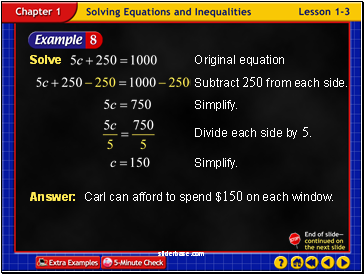Example 3-8b

Answer: Carl can afford to spend \$150 on each window.

Slide 62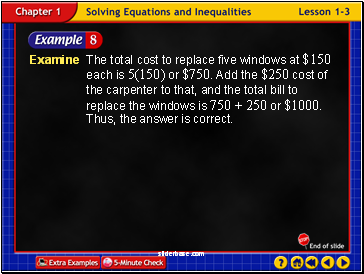Example 3-8c

Examine The total cost to replace five windows at \$150 each is 5(150) or \$750. Add the \$250 cost of the carpenter to that, and the total bill to replace the windows is 750 + 250 or \$1000. Thus, the answer is correct.

Slide 63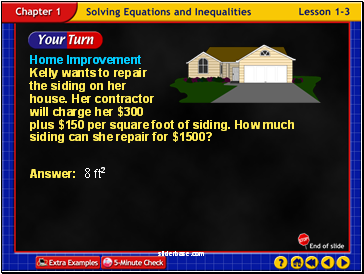Home Improvement Kelly wants to repair the siding on her house. Her contractor will charge her \$300 plus \$150 per square foot of siding. How much siding can she repair for \$1500?

Example 3-8d

Slide 64End of Lesson 3

Slide 65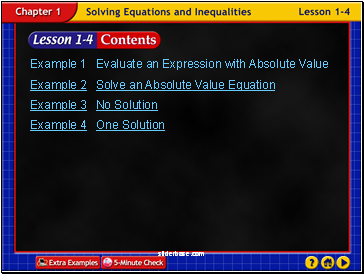Lesson 4 Contents

Example 1 Evaluate an Expression with Absolute Value

Example 2 Solve an Absolute Value Equation

Example 3 No Solution

Example 4 One Solution

Slide 66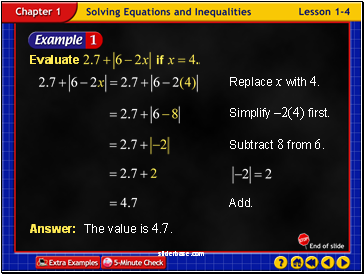Example 4-1a

Slide 67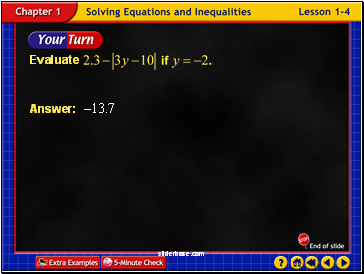Example 4-1b

Slide 68Example 4-2a

Slide 69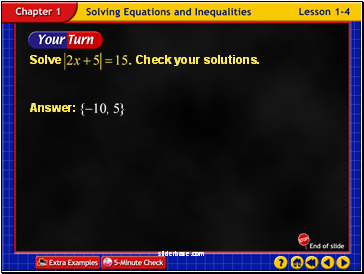Example 4-2b

Slide 70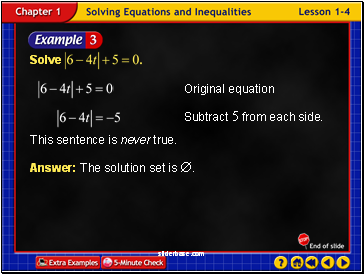Example 4-3a

Answer: The solution set is .

This sentence is never true.

Slide 71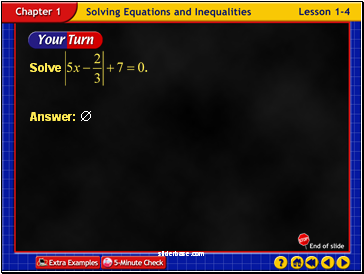Example 4-3b

Slide 72Example 4-4a

Slide 73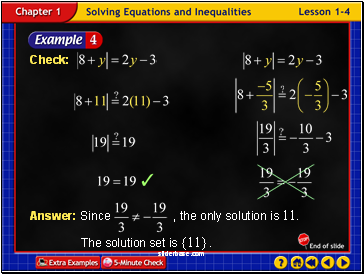Example 4-4b

Slide 74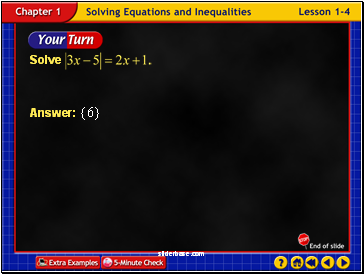Example 4-4c

Slide 75End of Lesson 4

Slide 76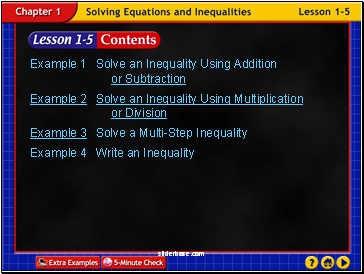Lesson 5 Contents

Example 1 Solve an Inequality Using Addition or Subtraction

Example 2 Solve an Inequality Using Multiplication or Division

Example 3 Solve a Multi-Step Inequality

Example 4 Write an Inequality

Slide 77

Go to page:
1  2  3  4  5  6  7  8